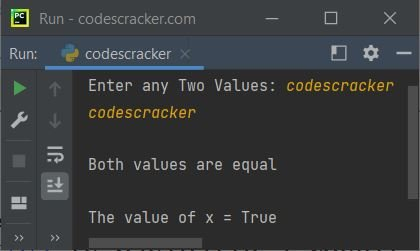# Python True Keyword

The "True" keyword in Python is basically a Boolean value. There are two boolean values: True and False. The "True" is basically a result of:

• a comparison operation
• or any other logical expression whose value evaluates in the form of True or False.

For example:

```a = 10
b = 20
c = 10

print(a==c)
print(a<b)```

The output is:

```True
True```

## Python True keyword example

True is the result of a boolean operation. That is, if the expression(s) of a boolean operation evaluate to be True, then the condition evaluates to be True. Here is an example that demonstrates it:

```print("Enter any Two Values: ", end="")
a = input()
b = input()

x = a==b
if x:
print("\nBoth values are equal")
else:
print("\nBoth values are not equal")

print("\nThe value of x =", x)```

The snapshot given below shows the sample run of the above program, with user input "codescracker" as the first and second values:### Advantages of the True keyword in Python

• It is a built-in keyword in Python, meaning it can be used without the need for additional definition or import statements.
• It is straightforward to comprehend and use in programming, particularly in conditional statements, as it represents a condition as being true.
• It is a fundamental component of Python's built-in logic, enabling more complex programming and algorithm design.

### Disadvantages of the True keyword in Python

• Because "True" is case-sensitive, "true" would be rejected as a boolean value.
• If used incorrectly, "True" can be misused in programming, such as in a loop without an exit condition, which can lead to an infinite loop.
• The "True" keyword can be ambiguous in certain cases, such as when dealing with empty containers; an empty container evaluates to "False,", while a non-empty container evaluates to "true."

The "True" keyword should be used with caution and intelligence to avoid errors and ambiguity in programming. The "True" keyword enables more intricate logical operations and algorithm design.

Python Online Test

« Previous Tutorial Next Tutorial »